### 明确概念

• ”前缀”和”后缀”：“前缀”指除了最后一个字符以外，一个字符串的全部头部组合；”后缀”指除了第一个字符以外，一个字符串的全部尾部组合。例如”ABCD”的前缀为[A, AB, ABC]，后缀为[BCD, CD, D].
• 最大公共前后缀：基于前缀，和后缀概念，在一个字符串中，最长的相同的前缀和后缀称为最长公共前后缀，有的称之为最长首尾串。
• Next[]数组：Next数组主要用来存放最大公共前后缀的长度值，此值定义为K值，即Next[]数组存储一组k值

### 开始匹配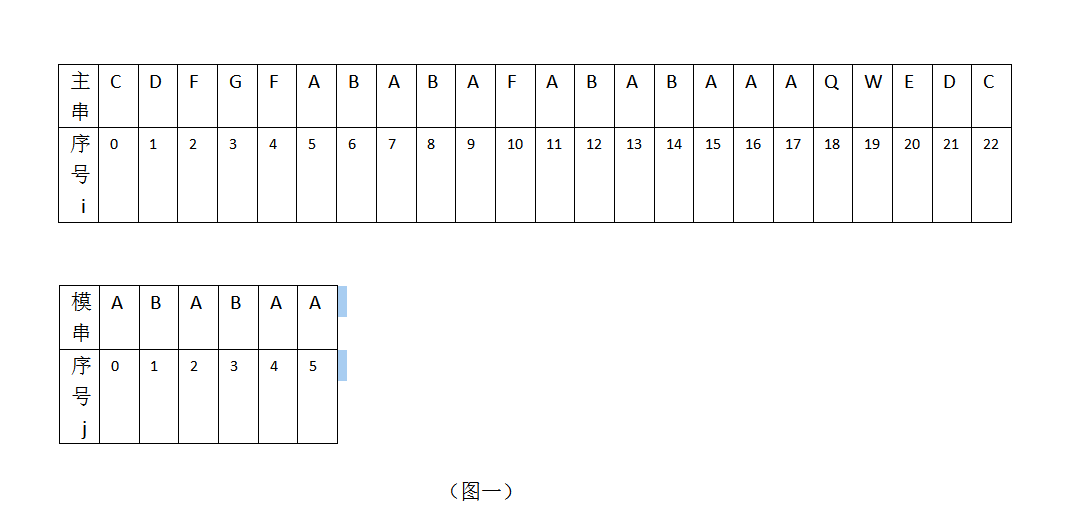• 模式串下一匹配位：定义为k，即下一次匹配时，主串i不变，模式串的新j值为j=k,即S[i]与T[k]进行比较。
• K如何取值：k=next[j-1]，为何用next[j-1]标识，之所以这样是为了更好的理解，j-1的含义是当j=5，i=10，T!=S时，需要取模式串”ABABAA”的next[]数组的第j-1=4位的数组值，此时Next[]={0,0,1,2,3,1}”，在此next[]数组中，next=3，即k=3。
• 注:还可以用另一个方法计算k，模式串j前面（不包括j），即从位置0开始，到4(j-1=4)结束的字符串”ABABA”的最长公共前后缀为”ABA”，它的长度值为3，即k=3，如下图：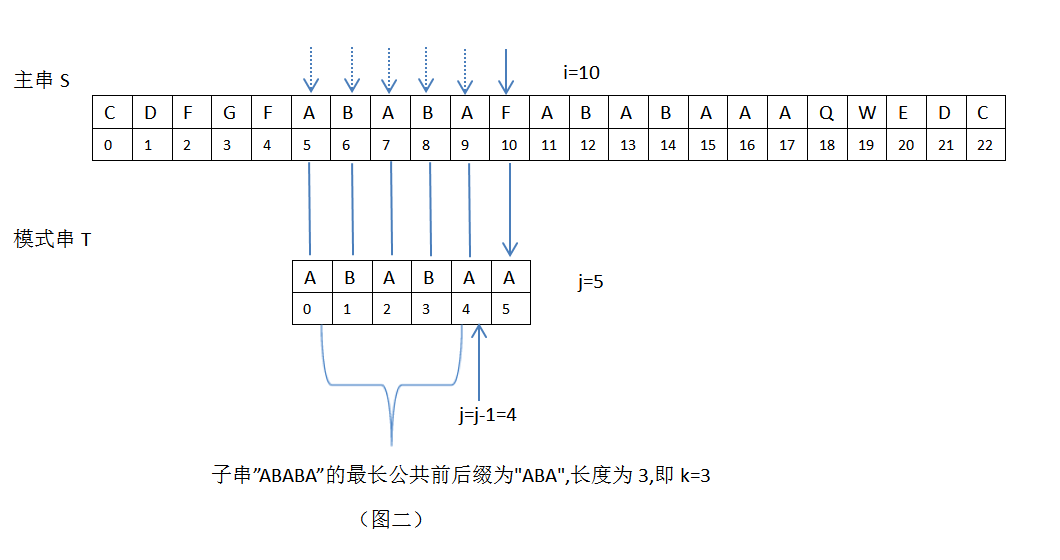• 模式串T向右滑动的最大距离：定义为m，m=j-k（当j>0时，j为模式串的序号）

• 字符串模式匹配时存在的两种情况：

1.当模式串j=0时，即T与主串Si且T!=S[i]时，主串S位置加1，T向右滑动1位，此时j=0，T将与主串Si继续比较。

2.当模式串j>0时，即T[j]与主串Si且T[j]!=S[i]时，此时会用到KMP算法。

### 举例KMP算法

1.初始时，i =0，j=0；S=”C”，T=”A”，S[i]!=T[j]，匹配失败，此时需要主串S位置加1，模式串T向右移动1位。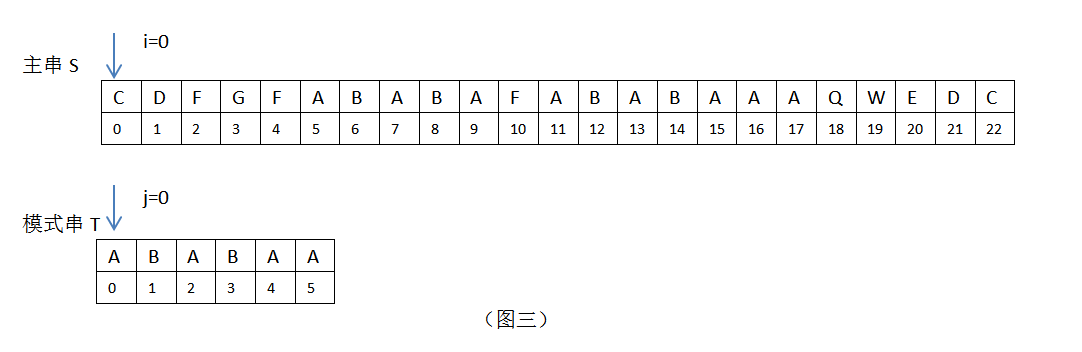2.当 i =1,2,3,4时，均匹配失败；此时需要主串S位置加1，模式串T向右移动1位。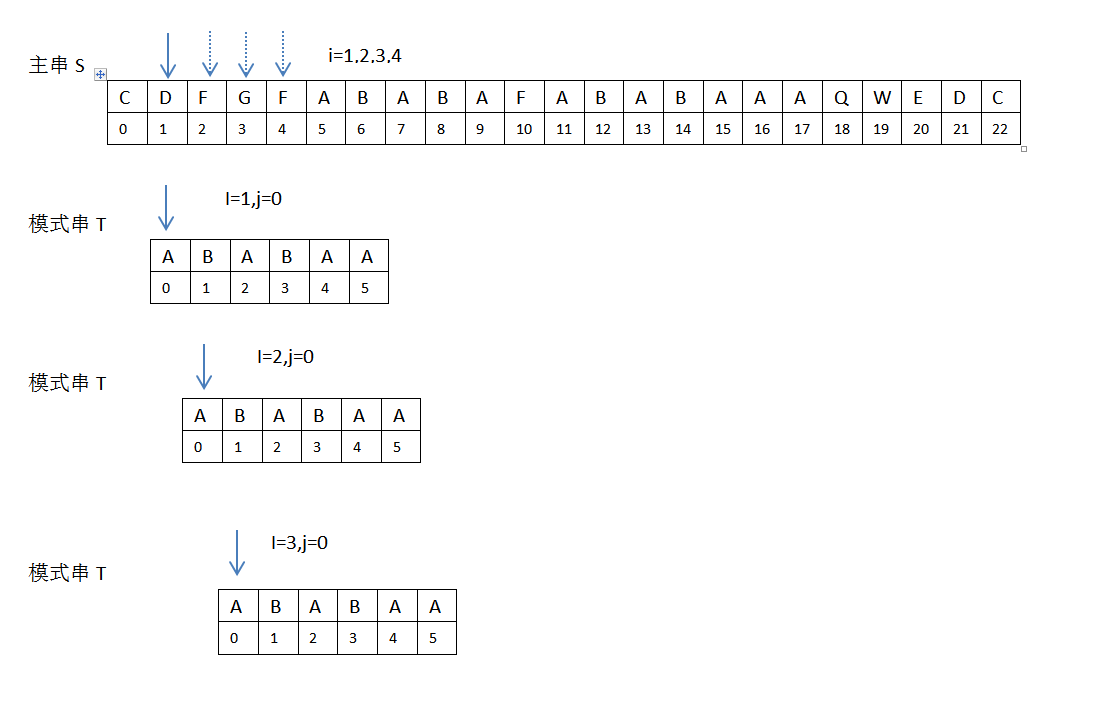3.当i=5,6,7,8,9，j=0,1,2,3,4时，匹配成功，此时主串S位置加1，模式串T位置加1位。4.当i=10，j=5时，匹配失败，S=“F”， T=“A”，S!=T，见下：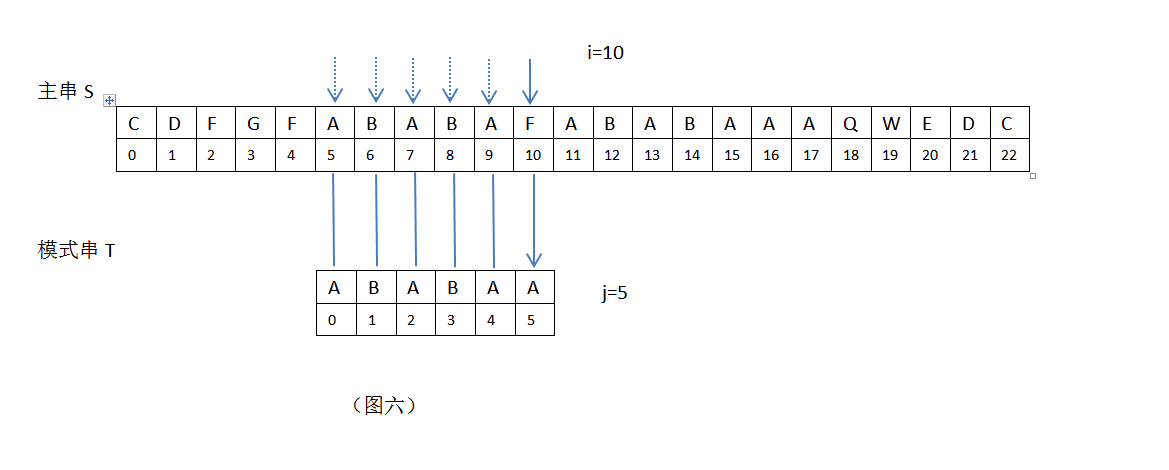5.在上图，i=10，j=5，接下来继续匹配时，需要主串中S[i]位置不变（i=10），模式串位置变为T[k]，现在需要求k值。根据公式k=next[j-1]，需要取模式串”ABABAA”的next[]数组的第j-1=5-1=4位的数组值，此时Next[]={0,0,1,2,3,1}”，在此next[]数组中，next=3，即k=3（j前面字符串”ABABA”的最长公共前后缀为”ABA”，它的长度值为3，即k=3）；此时，根据KMP算法，i=10不变，模式串T向右滑动m=j-k=5-3=2位，模式串新的j值为j=k=3，见下：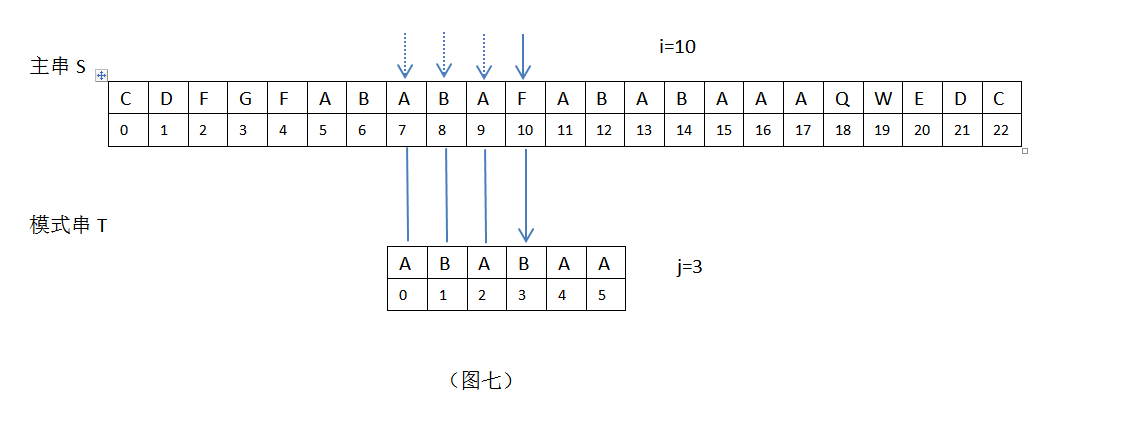6.在上图，i=10，j=3，S[i]=S=”F” ，T[j]=T=”B”，S!=T，接下来继续匹配时，需要主串中S[i]位置不变（i=10），模式串位置变为T[k]，现在需要求k值。根据公式k=next[j-1]，需要取模式串”ABABAA”的next[]数组的第j-1=3-1=2位的数组值，此时Next[]={0,0,1,2,3,1}”，在此next[]数组中，next=1，即k=1（j前面字符串”ABA”的最长公共前后缀为”A”，它的长度值为1，即k=1）；此时，根据KMP算法，i=10不变，模式串T向右滑动m=j-k=3-1=2位，模式串新的j值为j=k=1，见下：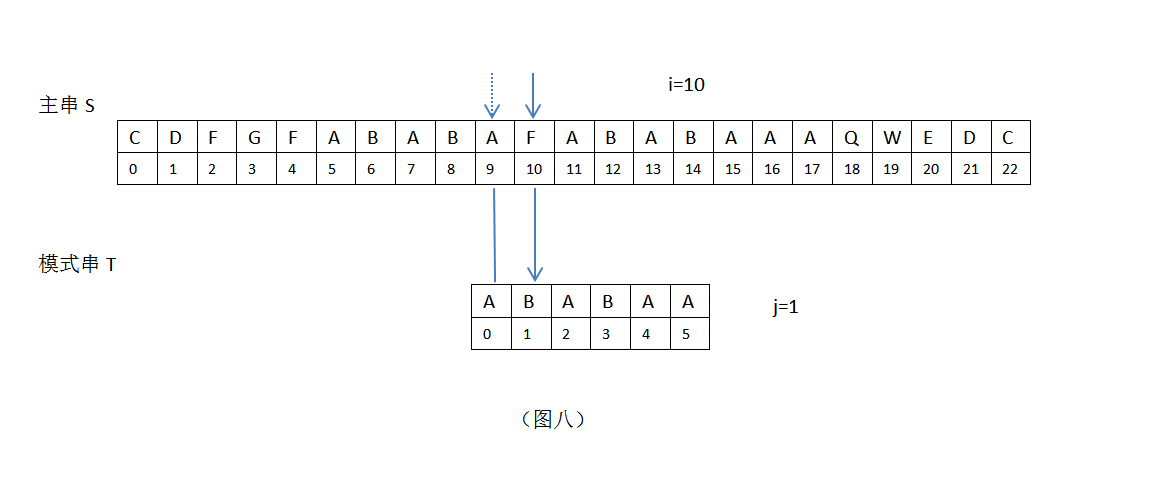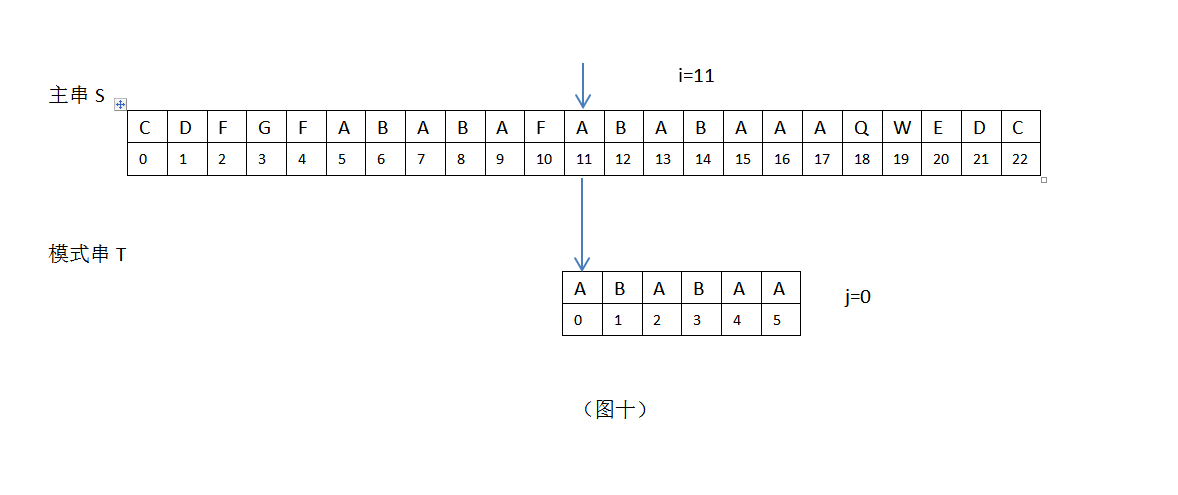### Python版代码

-------　本文结束　　感谢您的阅读　-------
(>看完记得五星好评哦(●'◡'●)<)